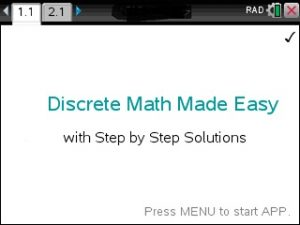## Step by Step Discrete & Finite Math app for the Ti-Nspire CAS CXOverview and Examples at https://youtu.be/8jdUbPRnijs

The most comprehensive Discrete Math APP for calculators.

Step by Step……

• Linear Programming
• Marginal Analysis, Demand Analysis
• Markoff Chains
• Simplex Algorithm
• Probabilities
• Combinatorics
• Functions
• Vector and Matrices
• Solve Equations
• Logic with Set Theory, Truth Tables
• Easy to use. Just plug in the equation and the correct answer shows.
Posted on Categories discrete math

## Step by Step Linear Algebra app for the Ti-Nspire CAS CXOverview and Examples : https://youtu.be/uqIRhvqqVDE

Perform 30+ Matrix Computations such as A+B, A-B, k*A, A*B, B*A, A-1, det(A), Eigenvalues, LU and QR – Factorization, Norm, Trace.

• Step by Step – Simplex Algorithm.
• Step by Step – Gaussian Elimination.
• Step by Step – Find Inverse
• Step by Step – Find Determinant
• Step by Step – Row Echelon and Reversal (REF and RREF)
• Step by Step – Gauss and Gauss Jordan Elimination
• Step by Step – Cramer Rule
• Step by Step – EigenValues and EigenVectors.
• Step by Step – Square Root Matrix
• Solve any n by n system of equations.
• Rotation Matrices, Magic Squares and much more.
• Step by Step – Solve AX=B
• Step by Step – OrthoNormal Basis
• Step by Step – Range, Kernel
• Nullity, Null-, Row- and ColumnSpace Basis.
• Cross and Dot Product, UnitVector, Angle between Vectors
• Projection A onto B, Distance A to B, etc …
Posted on Categories linear algebra

## Step by Step Trigonometry app for the Ti-Nspire CX available nowWatch this Youtube Video at https://youtu.be/nvvWKzVBgfA

STEP BY STEP – Solve any 90oand non 90o Triangle.Just enter 2 sides and an angle, 3 sides or 1 side and 2 angles to view each step until triangle is solved.

• This app is intelligent enough to automatically apply the Law of Sine or the Law of Cosine and other basic Triangle Rules to solve any triangle STEP by STEP.
• It even notifies if triangle cannot be solved or solutions are ambiguous.
• Read the Trig-Theorems and Identities.
• Evaluate Trig Functions and set up Trig-Models.
• Master the Unit-Circle and its Coordinates.
• Compute Sectors and Arcs.
• Convert Degree to Radian and vice versa.
• STEP BY STEP – Given Sin Cos or Tan find the remaining ratios in a 90 degree triangle.
• Right Triangle Checker: Check For Right Angle given 3 points
• Trig Identity Checker
• Convert Degrees(decimals) to Degrees, Minutes, Seconds
• Convert Cartesian Coordinates to Polar Coordinates and vice versa.

Watch how to use the app when doing Trig homework at http://<iframe width=”460″ height=”335″ src=”https://www.youtube.com/embed/RSHEQztJdw4″ frameborder=”0″ allowfullscreen></iframe>

Posted on Categories trigonometry

## Step by Step PreCalculus app for the Ti-Nspire CAS CX available nowWatch this Youtube Video for an overview and examples at https://youtu.be/cRIs3oUicrw

Complete and Comprehensive.

Step by Step Solutions to : Functions (Polynomial, Exponential, Logarithmic and Trigonometric Functions), Trigonometry, Statistics, Discrete Mathematics, Algebra, Matrices, Complex Numbers, Sequences, Introductory Calculus.

Watch this Video on using the app doing PreCalculus homework at https://youtu.be/B9bYtG52XE4

## Step by Step Algebra app for the Ti-Nspire – Watch this Video at https://youtu.be/Q0uXzdzzHog

Step by Step Algebra App for the Ti-NSpire at www.tinspireapps.com. It covers the ENTIRE Algebra curriculum: Algebra, Functions and their Analysis, Trig, Geometry, Complex Numbers, Matrices, Exponential/Logarithmic Functions, Probabilities, Combinatorics, Sequences, Induction, Sigma Notation, etc

• Step by Step – Complete the Square
• Step by Step – Partial Fractions
• Step by Step – Complex Numbers
• Step by Step – Powers
• Step by Step – Roots
• Step by Step – Synthetic Division
• Step by Step – Radicals
• Logarithm and Exponential Solver
• Rewrite Logarithms into their Exponential Form and back: log_b(y)=x <–> y=b^x
• Expand and Condense Logarithms Step by Step
• Rule of 72, Change of Logarithm base
• Effective Interest rates
• Euler Number as Limit Definition.
• Money Growth Solver

## Step by Step Differential Equations app for the Ti-Spire – Watch this Video at https://youtu.be/7lH9GrSv2jAO8

Great News: Step by step Differential Equations App for the TiNspire is now available for download at www.tinspireapps.com . It includes:

• Step by Step Linear Diff Eqn
• Step by Step Variation of Parameter
• Step by Step Linear Undetermined Coefficients
• Step by Step Separation of Variables
• Step by Step Exact and Non-Exact Diff Eqns
• Step by Step Laplace Transforms
• Step by Step Wronskian
• Step by Step Cauchy Euler Diff Eqns
• Step by Step Numerical Solutions : Euler, Runge Kutta
• Step by Step Homogeneous Diff Eqns. and Characteristic Polynomial
• Step by Step Eigenvalues and Eigenvectors
• Step by Step Logistic Growth Diff. Equation
• and much more.

Posted on Categories differential equation

## Step by Step Chemistry app now available for the TI-Nspire CX CAS

TI-Nspire users:

Step by Step Chemistry problems involving

Period Table of Elements,

Equation Balancing,

Stoichiometry,

Theoretical and %-Yield

Limiting Reagent

Hess Law

Molecule Mass Analysis

Gas Laws,

Moles, Mass, Density, Volume, Weight etc

Free trials.